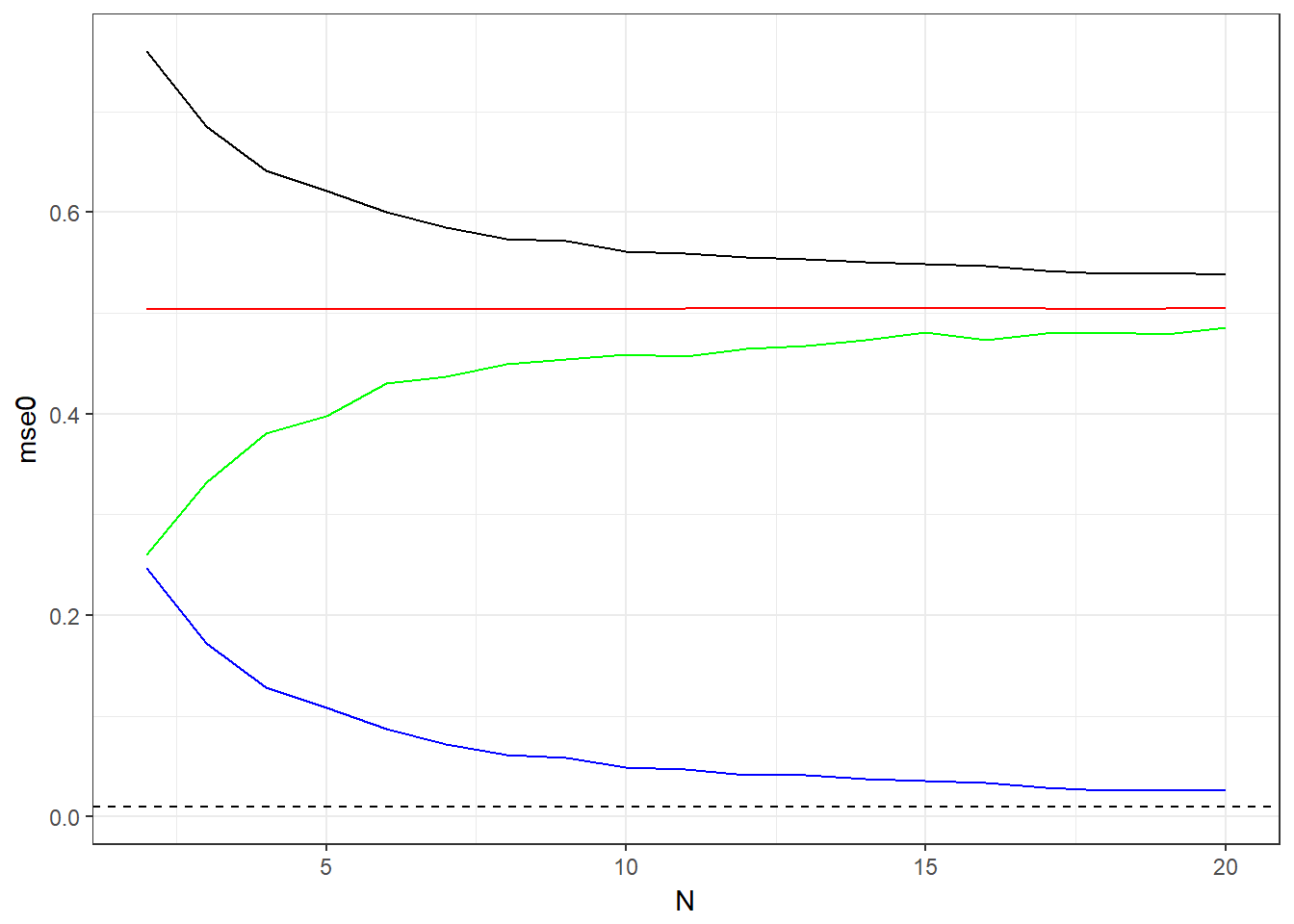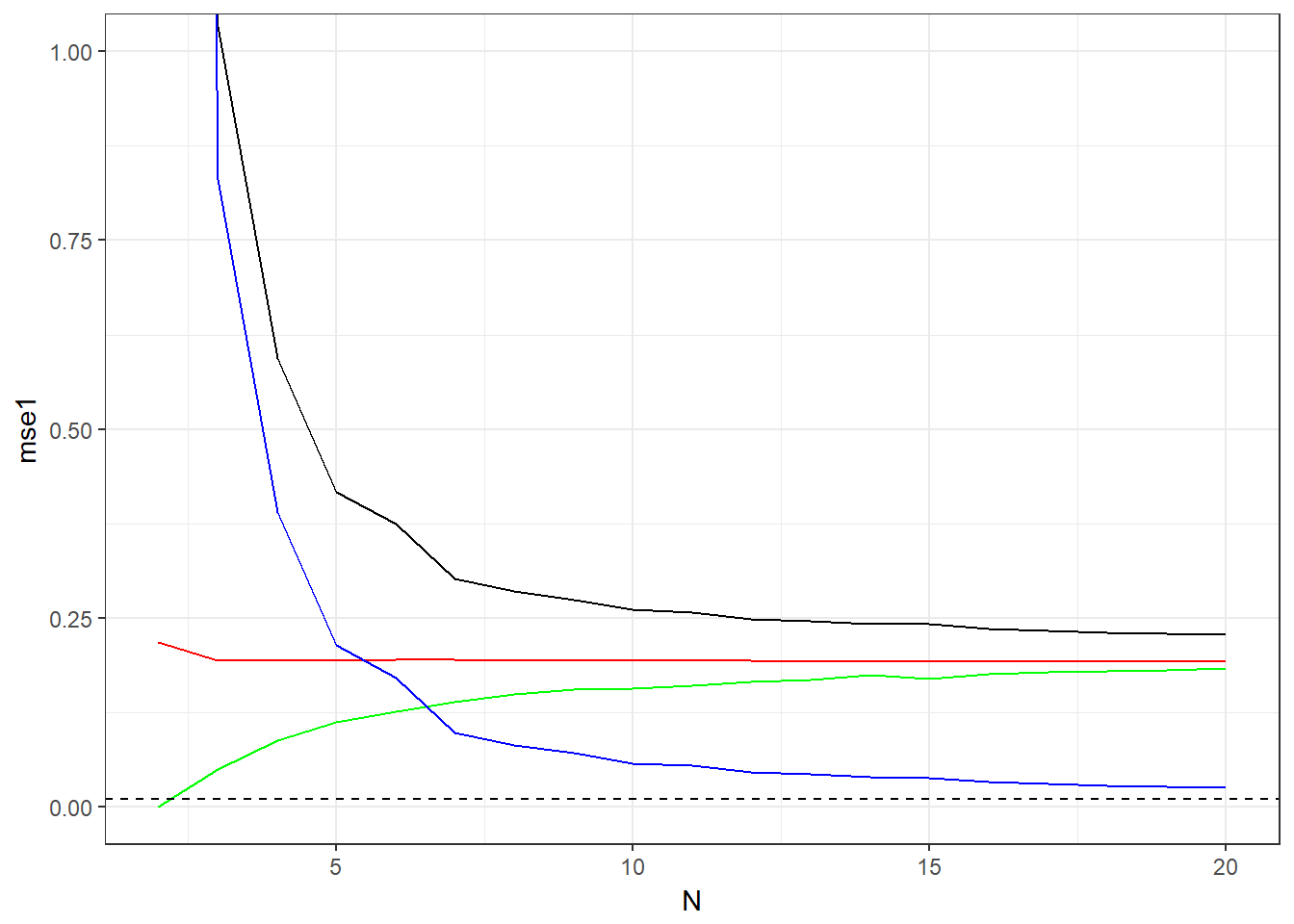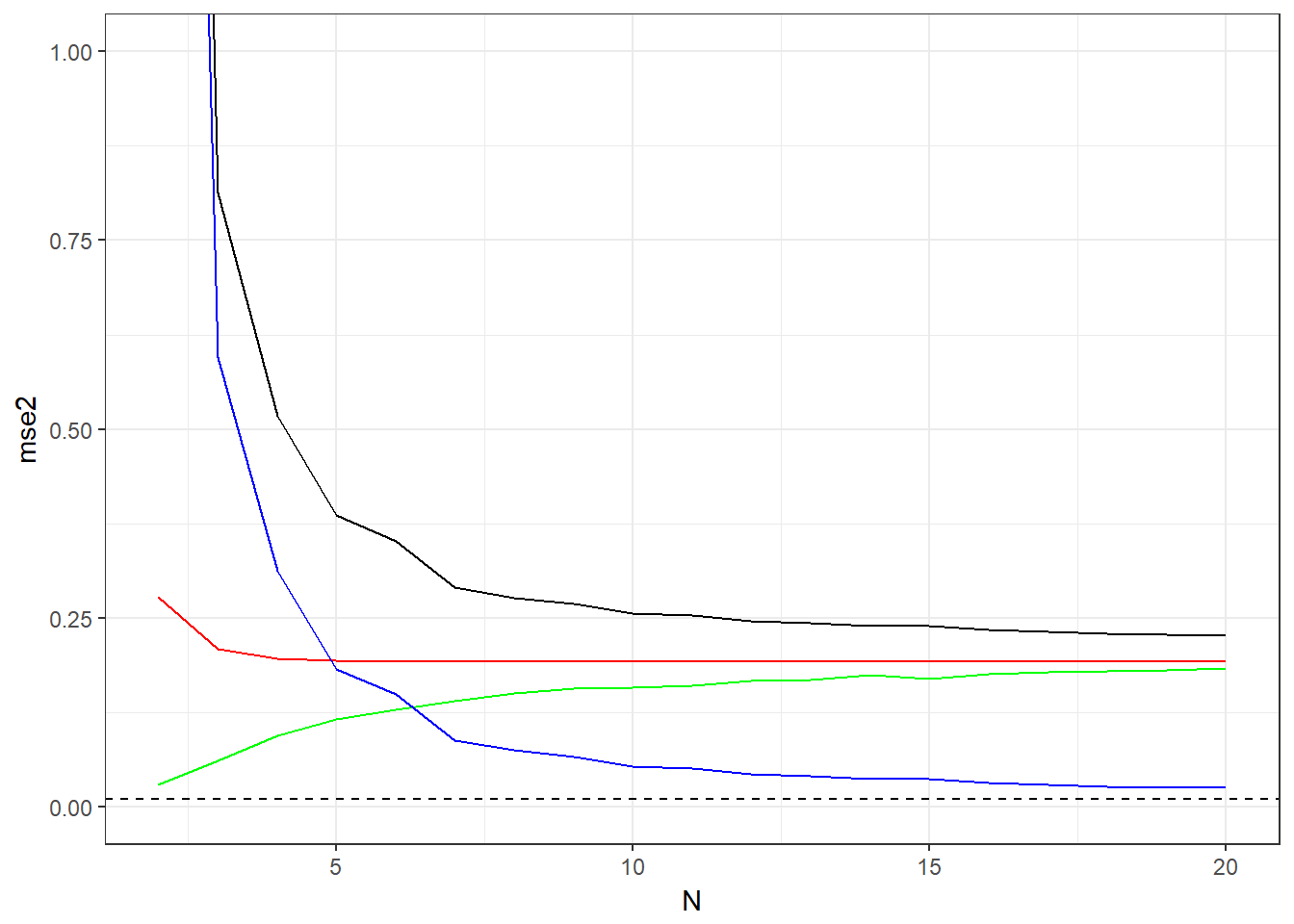### Problem 4.17

\begin{align*} \hat{E}_{in}(\mathbf{w}))&=\mathbb{E}_{\boldsymbol{\epsilon}_1\cdots\boldsymbol{\epsilon}_N}\left[\frac{1}{N}\sum\limits_{n=1}^N(\mathbf{w}^T\hat{\mathbf{x}}_n-y_n)^2\right]\\ &=\mathbb{E}_{\boldsymbol{\epsilon}_1\cdots\boldsymbol{\epsilon}_N}\left[\frac{1}{N}\sum\limits_{n=1}^N(\mathbf{w}^T(\mathbf{x}_n+\boldsymbol{\epsilon}_n)-y_n)^2\right]\\ &=\mathbb{E}_{\boldsymbol{\epsilon}_1\cdots\boldsymbol{\epsilon}_N}\left[\frac{1}{N}\sum\limits_{n=1}^N(\mathbf{w}^T\mathbf{x}_n+\mathbf{w}^T\boldsymbol{\epsilon}_n-y_n)^2\right]\\ &=\mathbb{E}_{\boldsymbol{\epsilon}_1\cdots\boldsymbol{\epsilon}_N}\left[\frac{1}{N}\sum\limits_{n=1}^N(\mathbf{w}^T\mathbf{x}_n-y_n)^2+2(\mathbf{w}^T\mathbf{x}_n-y_n)\mathbf{w}^T\boldsymbol{\epsilon}_n+(\mathbf{w}^T\boldsymbol{\epsilon}_n)^2\right]\\ &=E_{in}+\frac{1}{N}\mathbb{E}_{\boldsymbol{\epsilon}_1\cdots\boldsymbol{\epsilon}_N}\left[\sum\limits_{n=1}^N(\mathbf{w}^T\boldsymbol{\epsilon}_n)^2\right]\\ &=E_{in}+\frac{1}{N}\sum\limits_{n=1}^{N}\mathbb{E}\left[(\mathbf{w}^T\boldsymbol{\epsilon}_n)^2\right] \end{align*} Since $$\mathbf{w}^T\sigma^2_x\mathbf{I}\mathbf{w}=$$Var$$[\mathbf{w}^T\boldsymbol{\epsilon}_n]=\mathbb{E}[(\mathbf{w}^T\boldsymbol{\epsilon}_n)^2]-\mathbb{E}[\mathbf{w}^T\boldsymbol{\epsilon}_n]^2=\mathbb{E}[(\mathbf{w}^T\boldsymbol{\epsilon}_n)^2]$$ we have \begin{align*} E_{in}+\frac{1}{N}\sum\limits_{n=1}^{N}\mathbb{E}\left[(\mathbf{w}^T\boldsymbol{\epsilon}_n)^2\right]&=E_{in}+\frac{1}{N}\sum\limits_{n=1}^{N}\mathbf{w}^T\sigma^2_x\mathbf{I}\mathbf{w}\\ &=E_{in}+\sigma^2_x\mathbf{w}^T\mathbf{I}\mathbf{w} \end{align*}

which is the augmented error for linear model with Tikhonov regularizer. Here $$\Gamma=\sigma_x\mathbf{I}$$ and $$\lambda=N$$.

### Hypothesis using ridge regression:

hr <- function(data) {
EinR = function(w,data) {
#function Ein + lambda w^2
sum( (data$y -w -w*(data$x-mean(data$x))/sd(data$x) )^2 )+0.5*(w^2);
}

#opitmization
w = optim(c(0.1,0.1),EinR, data = data)$par; #unnormalize w = w - w*mean(data$x)/sd(data$x) w = w/sd(data$x)

return(w)
}

## Learning Curves:

Code to calculate these are the same as in the LearningCurve.Rmd demo. Just copy all the lines for h1 and increase the index.

Comparing the bias (red), we see that Ridge regression increases the bias compared to Linear regression, and lowers the bias compared to the constant. Comparing the variance (blue), we see that Ridge regression lowers the variance compared to linear regression, and increases the variance compared to the constant. The shape of the ridge regression graph is similar to that of the linear regression here as $$\lambda$$ is small. If we take $$\lambda$$ to be $$0$$ or very large, we get graphs that is exactly the linear regression one (for $$\lambda=0$$) and a very similar one to the constant one when $$\lambda$$ is very large.

For linear regression, we note that the Ein can start from zero when N is low N is <= to the dvc. Ridge doesnt have this as it allows us to have dvc that is “continuous”.

### H0: Constant### H1: Linear### H2: Ridge